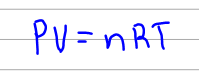Chemistry Practice Problems Chemistry Gas Laws Practice Problems Solution: An automobile tire has a maximum rating of 38.0 ps...

🤓 Based on our data, we think this question is relevant for Professor N/A's class at Ryerson University.

# Solution: An automobile tire has a maximum rating of 38.0 psi (gauge pressure). The tire is inflated (while cold) to a volume of 11.8 L and a gauge pressure of 36.0 psi (Note: The gauge pressure is the difference between the total pressure and atmospheric pressure. In this case, assume that atmospheric pressure is 14.7 psi) at a temperature of 12.0°C. While driving on a hot day the tire warms to 65.0°C and its volume expands to 12.2 L. What is the pressure (psi)  in the tire after warming on a hot day? Express your answer in pound-force per square inch to 3 significant figures.

###### Problem

An automobile tire has a maximum rating of 38.0 psi (gauge pressure).

The tire is inflated (while cold) to a volume of 11.8 L and a gauge pressure of 36.0 psi (Note: The gauge pressure is the difference between the total pressure and atmospheric pressure. In this case, assume that atmospheric pressure is 14.7 psi) at a temperature of 12.0°C. While driving on a hot day the tire warms to 65.0°C and its volume expands to 12.2 L.

What is the pressure (psi)  in the tire after warming on a hot day? Express your answer in pound-force per square inch to 3 significant figures.

###### Solution

For this problem we would have to use the ideal gas law to solve for the pressure:View Complete Written Solution

Chemistry Gas Laws

Chemistry Gas Laws

#### Q. The ideal gas law (PV = nRT) describes the relationship among pressure P. volume V, temperature T. and molar amount n. When some of these variables ar...

Solved • Tue Jul 24 2018 11:41:01 GMT-0400 (EDT)

Chemistry Gas Laws

#### Q. If a fixed amount of a gas is at a pressure of 2.71 atm and has a volume of 85.3 L at a temperature of 173.4 K, what is the final temperature of the g...

Solved • Tue Jul 24 2018 09:42:40 GMT-0400 (EDT)

Chemistry Gas Laws

#### Q. A sample of nitrogen gas at 298 K and 745 torr has a volume of 37.42 L. What volume will it occupy if the pressure is increased to 894 torr at constan...

Solved • Tue Jul 17 2018 15:48:05 GMT-0400 (EDT)

Chemistry Gas Laws

#### Q. Under constant-pressure conditions a sample of hydrogen gas initially at 63.00°C and 8.90 L is cooled until its final volume is 4.10 L. What is its fi...

Solved • Tue Jul 17 2018 14:09:34 GMT-0400 (EDT)